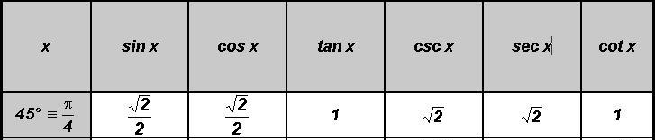POINT OF INTEREST 1
NUMERIC VALUES OF THE TRIGONOMETRIC RATIOS OF SPECIAL ANGLESWe will derive the numeric values of the six trigonometric ratios of the special angles 30o, 45o, 60o using right triangles and the following definitions.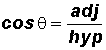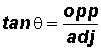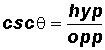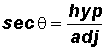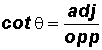Let's derive the values of the six trigonometric ratios of the 30oangle.

We will use the 30-60-90 triangle. Its sides are in the proportions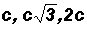, where 2c is the length of the hypotenuse of the triangle.For simplicity's sake, we will let c = 1, although, c could take on any value and we would still get the same answers!!!

Using the definition of the trigonometric ratios, please convince yourself that the EXACT values of the six trigonometric ratios of the angle 30o are as follows: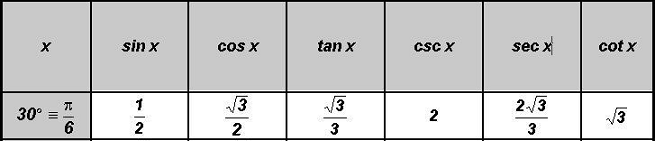Let's derive the values of the six trigonometric ratios of the 60o angle.

We will again use the 30-60-90 triangle.For simplicity's sake, we will let c = 1, although, c could take on any value and we would still get the same answers!!!

Using the definition of the trigonometric ratios, please convince yourself that the EXACT values of the six trigonometric ratios of the angle 60o are as follows: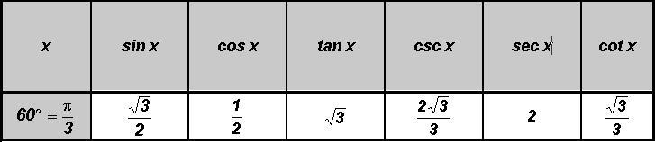Let's derive the values of the six trigonometric ratios of the 45o angle.

We will use the 45-45-90 triangle. Its sides are in the proportions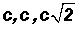, whereis the length of the hypotenuse of the triangle.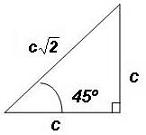For simplicity's sake, we will let c = 1, although, c could take on any value and we would still get the same answers!!!

Using the definition of the trigonometric ratios, please convince yourself that the EXACT values of the six trigonometric ratios of the angle 45o are as follows: